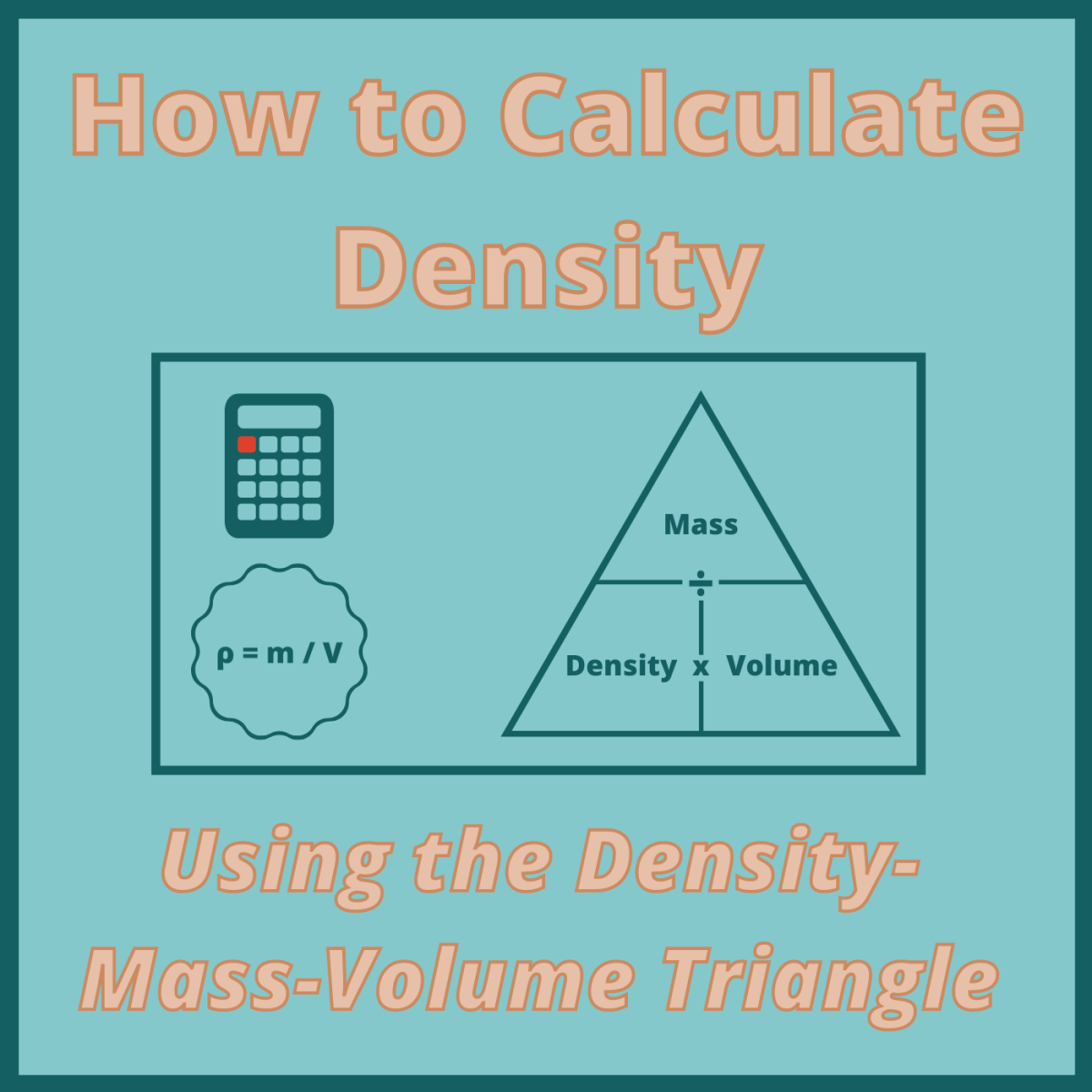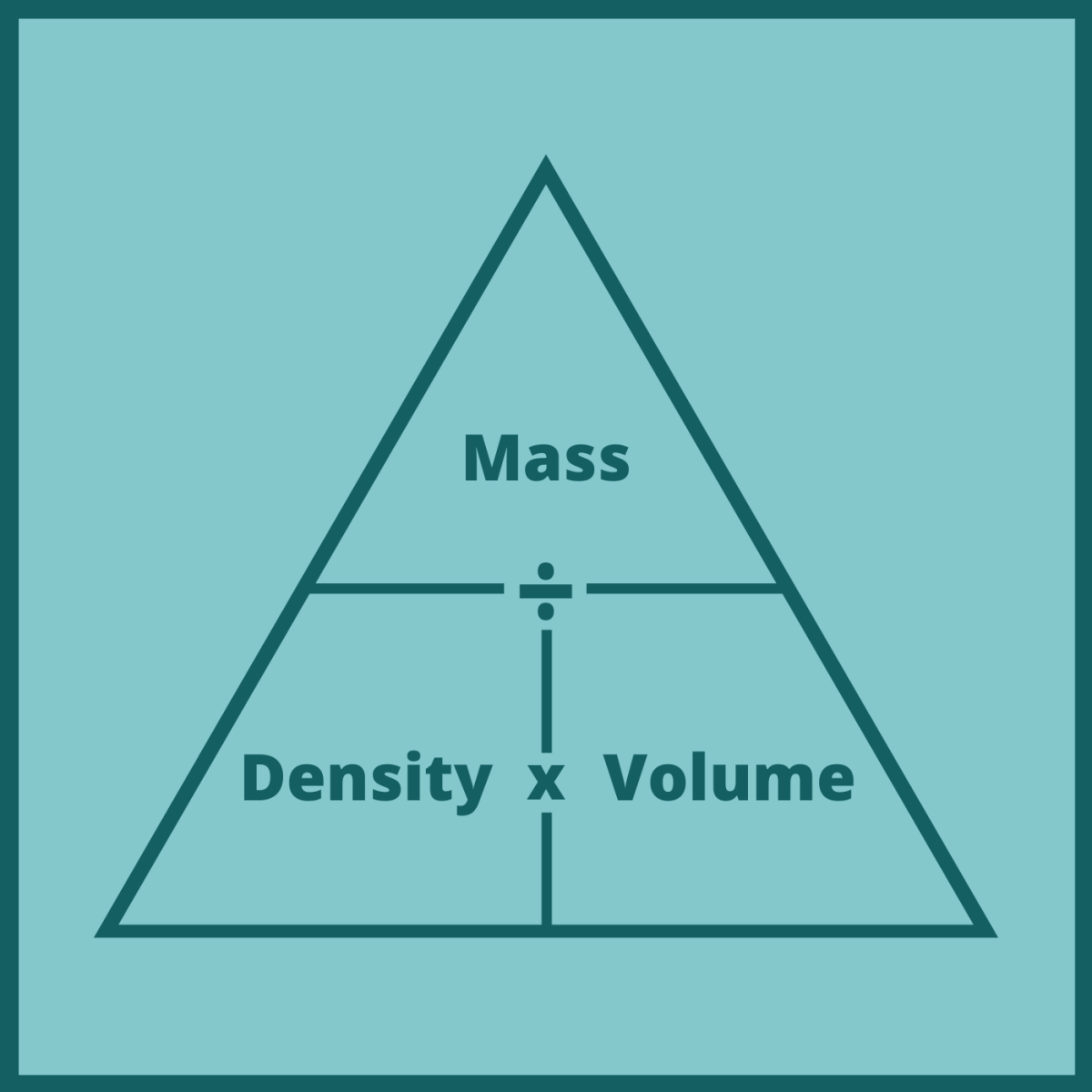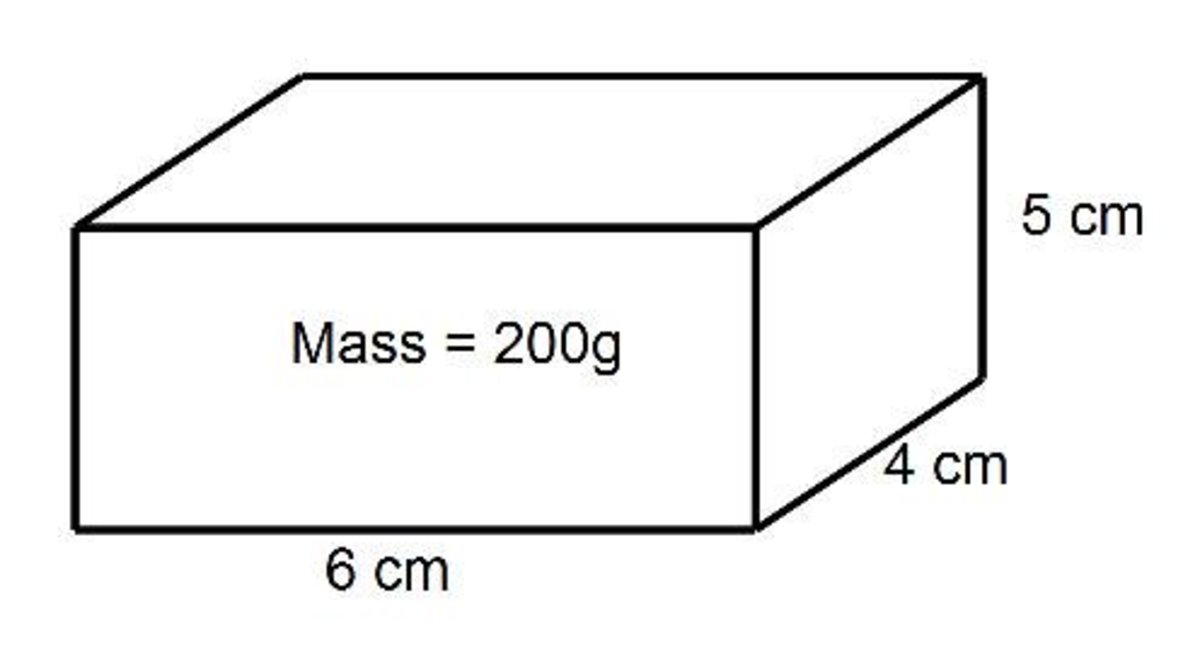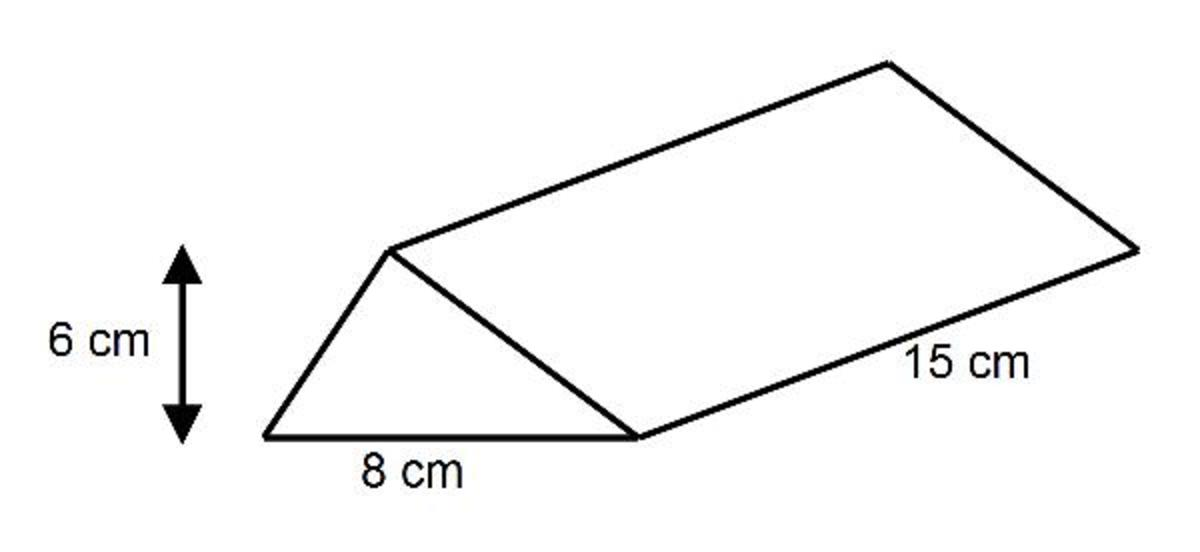Using the Density-Mass-Volume Triangle to Calculate Density

• Author:
• Updated date:

Mark, a math enthusiast, loves writing tutorials for stumped students and those who need to brush up on their math skills.Learn how to calculate the density, mass, or volume of a solid object using this handy visual tool. Canva

What Is Density?

Density is a measure of how compact (dense) an object is. If an object has a greater density than water, the object will sink in water. If it has a lower density than water, it will float.

How Do You Work Out the Density of a Solid Object

To calculate an object's density, we'll need to know the object's mass and volume. We can then plug those values into the following equation to calculate the object's density:

Density = Mass / Volume

This formula is often written using the following symbols:

• ρ: density
• m: mass
• V: volume

Using these symbols as variables, our formula now looks like this:

ρ = m / VThe mass-density-volume triangle helps us visualize the relationships between these three variables.Canva

What Is the Density-Mass-Volume Triangle?

The density-mass-volume triangle (pictured above) is a visual tool that we can use to figure out how to calculate the density, mass, or volume of a solid object if we know the two remaining values. The triangle is divided into three parts, with density occupying the top portion and mass and volume occupying the bottom two portions.

The positions of each element of the triangle show us how they relate to one another through the formula above (Density = Mass / Volume, or ρ = m / V).

How to Calculate Density

If we want to calculate density, we simply cover up density in the triangle and see what remains. Since mass is above volume, we divide mass by volume to get density.

Density = Mass / Volume

ρ = m / V

How to Calculate Mass

If we want to calculate mass, we simply cover up mass in the triangle and see what remains. Since density and volume are side by side, we multiply density by volume to get mass.

Mass = Density * Volume

m = ρ * V

How to Calculate Volume

If we want to calculate volume, we simply cover up volume in the triangle and see what remains. Since mass is above density, we divide mass by density to get volume.

Volume = Mass / Density

V = m / ρ

Example Problems With Processes and Solutions

Let’s take a look at some example problems and solve them using the density-mass-volume triangle explained above.In this first example, we need to calculate the density of this rectangular prism, or cuboid.

Example 1

A solid rectangular box has a length of 6 cm, a width of 4 cm, and a height of 5 cm. Work out the density of the box if the mass of the box is 200 g.

This question asks us to work out the density. Therefore, we'll need the formula for density (Density = Mass / Volume).

However, the question states the mass of the box but doesn’t state the volume, so we'll need to work out the volume of the box before we can work out the density.

Since the box is a cuboid, the volume can be found by multiplying lengths of the three side lengths together:

Volume = Length * Width * Height

V = 6 * 4 * 5

V = 120 cm³

Now that we have the volume, the density can be calculated:

Density = Mass / Volume

ρ = m / V

ρ = 200 / 120

ρ = 1.67 g/cm³In this second example, we need to calculate the mass of this triangular prism.

Example 2

Work out the mass of this triangular prism if the density is 3 g/cm³.

This time, we are asked to work out the mass, so we'll need the formula for mass (Mass = Density * Volume).

In this question, we are given the density but not the volume, so let’s begin by calculating the volume of the triangular prism. The cross sectional area of the prism can be found by using the formula Area = ½ * (Base * Height).

A = ½ * (6 * 8) = 24 cm²

The volume of the prism can now be found by multiplying this area by the length:

ρ = 24 * 15

ρ = 360 cm³

Now that we have the volume, we can work out the mass of the triangular prism:

Mass = Density * Volume

m = ρ * V

m = 3 * 360

m = 1080 g (or 1.08 kg)

This content is accurate and true to the best of the author’s knowledge and is not meant to substitute for formal and individualized advice from a qualified professional.

Question: A container is a rectangular prism. Its dimensions are 50 cm X 40 cm X 10 cm. If it filled with water, what is the mass of the water?

Answer: First, work out the volume of the rectangular prism by multiplying the three dimensions together, 50 multiplied by 40 multiplied by 10 to give 20,000 cm^3. Now since 1cm^3 is the same as 1g of water the answer is 20,000g (or 20kg).

Question: How much mass in rectangular prism height of 100 and a length of 25 and width of 3?

Answer: First find the volume by multiplying the numbers together to give 7500. Now multiply this answer by the density of the rectangular prism to give the mass.

Question: How do you calculate the density of a cube?

Answer: First work out the volume of the cube by cubing the side length.

Then divide the mass (given in the question) by the volume.

Question: What is density?

Answer: Density can be worked out by dividing the mass by the volume.

Question: A wooden block is a rectangular prism. Its length is 8 cm, the length of one of the two perfect square sides is 2 cm, the mass of the wooden block on the electronic balance reads 150 grams, what is the density of the wooden block?

Answer: First work out the volume of the block which is 32 cm^3 (8 times 2 times 2).

Then divide 150 by 32 to give 4.6875 g/cm^3